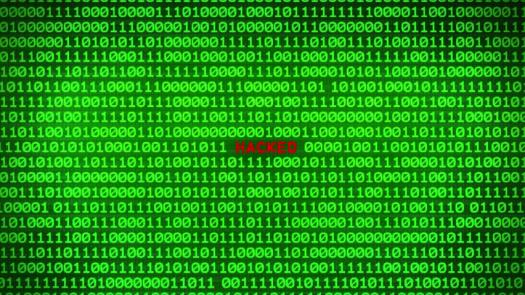# A Test On Kilobytes

Approved & Edited by ProProfs Editorial Team
The editorial team at ProProfs Quizzes consists of a select group of subject experts, trivia writers, and quiz masters who have authored over 10,000 quizzes taken by more than 100 million users. This team includes our in-house seasoned quiz moderators and subject matter experts. Our editorial experts, spread across the world, are rigorously trained using our comprehensive guidelines to ensure that you receive the highest quality quizzes.
| By Livyn
L
Livyn
Community Contributor
Quizzes Created: 347 | Total Attempts: 144,443
Questions: 10 | Attempts: 139SettingsWell, to buy one kilobyte nowadays is absurd and it's even impossible. This is as opposed to buying a yottabyte. That said, a kilobyte is like the far younger brother of terabytes and others. Nonetheless, we digress and we should instead go on with the quiz.

• 1.

### Which of the following best represent kilobytes?

• A.

10^6

• B.

10^5

• C.

10^4

• D.

10^3

D. 10^3
Explanation
The correct answer is 10^3. Kilobytes is a unit of digital information storage and it represents 1000 bytes. The prefix "kilo" in the metric system denotes a factor of 1000. Therefore, 10^3, which is equal to 1000, best represents kilobytes.

Rate this question:

• 2.

### How do you represent a kilobyte?

• A.

KE

• B.

KY

• C.

KT

• D.

K

D. K
Explanation
The correct answer is "K". In data storage, a kilobyte is represented by the letter "K". It is a unit of digital information that is equal to 1024 bytes. This notation is commonly used in computer science and information technology to represent data sizes.

Rate this question:

• 3.

### Technically, one kilobyte means

• A.

1020 bits

• B.

1020 bytes

• C.

1000 bits

• D.

1000 bytes

D. 1000 bytes
Explanation
One kilobyte technically means 1000 bytes. In computer systems, storage capacity is typically measured in bytes, where each byte represents 8 bits. Therefore, one kilobyte is equal to 1000 bytes, not 1020 bits or 1020 bytes.

Rate this question:

• 4.

### Which of the following uses kilobytes extensively?

• A.

Labels on hardisks

• B.

Speed of yottabyte

• C.

Calculating monthly internet traffic

• D.

Main memory

D. Main memory
Explanation
Main memory is the correct answer because kilobytes are commonly used to measure the capacity and size of computer memory. Main memory, also known as RAM (Random Access Memory), is used to store data and instructions that are currently being used by the computer's processor. Kilobytes are often used to quantify the amount of memory available in a computer system, as well as the size of individual files and programs that are loaded into memory. Therefore, main memory is the most appropriate option that extensively uses kilobytes.

Rate this question:

• 5.

### Who describes kilobytes as 1000 bytes?

• A.

The International Electronics Community

• B.

The International Electronics Commission

• C.

The International Electrotechnical Community

• D.

The International Electrotechnical Commission

D. The International Electrotechnical Commission
Explanation
The International Electrotechnical Commission (IEC) describes kilobytes as 1000 bytes. The IEC is an international standards organization that sets standards for electrical and electronic technologies. They have defined the prefixes for binary multiples, including kilo-, which represents 1000. This is in contrast to the International System of Units (SI), which defines kilo- as 1024. Therefore, the IEC's definition of kilobytes aligns with the decimal system commonly used in electronics and computer storage.

Rate this question:

• 6.

### 65,536 bytes of memory can be represented by

• A.

66K

• B.

65K

• C.

64K

• D.

63K

C. 64K
Explanation
65,536 bytes is equal to 64 kilobytes (KB) because 1 kilobyte is equal to 1024 bytes. Therefore, the correct answer is 64K.

Rate this question:

• 7.

### 1 MB in kilobytes is

• A.

1024^2

• B.

1020^2

• C.

1024^3

• D.

1020^4

A. 1024^2
Explanation
The correct answer is 1024^2 because 1 megabyte (MB) is equal to 1024 kilobytes (KB). The exponent of 2 indicates that the conversion is being done from bytes to kilobytes. Therefore, multiplying 1024 by itself (1024^2) gives the correct conversion of 1 MB to kilobytes.

Rate this question:

• 8.

### IEC used what to represent 1 kilobyte

• A.

Kiibytes

• B.

Kibibytes

• C.

Kibytes

• D.

Kiibibytes

B. Kibibytes
Explanation
The correct answer is Kibibytes. The International Electrotechnical Commission (IEC) uses the term "Kibibytes" to represent 1 kilobyte. This is a binary unit of digital information storage, equal to 2^10 bytes. It is used to differentiate from the decimal-based kilobyte, which is equal to 10^3 bytes. The IEC introduced this term to provide a more precise and accurate representation of data storage.

Rate this question:

• 9.

### Which one doesn't use the binary interpretation (kilobytes)?

• A.

AT&T

• B.

Verizon

• C.

Vodafone

• D.

Orange

B. Verizon
Explanation
Verizon is a telecommunications company that operates primarily in the United States. The question is asking which company does not use the binary interpretation for kilobytes. Kilobytes is a unit of digital information that is commonly used in computing. While AT&T, Vodafone, and Orange are all international telecommunications companies, Verizon is the only one that does not use the binary interpretation for kilobytes.

Rate this question:

• 10.

### Unformatted, the Shugart SA-400 5​1⁄4-inch floppy disk held how much?

• A.

639K

• B.

256K

• C.

110K

• D.

128KBack to top